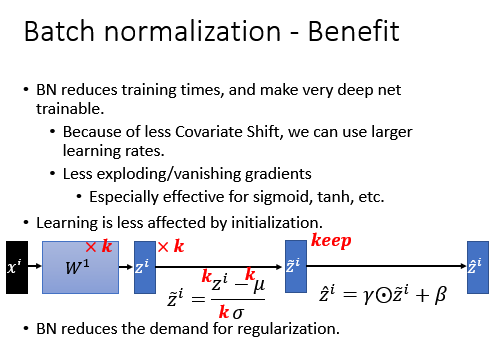# 经典算法：Batch Normalization

## Feature Scaling 特征缩放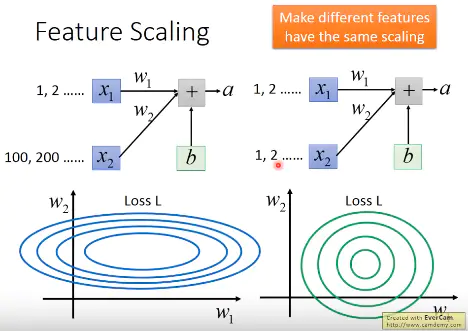## 经典的Feature Scaling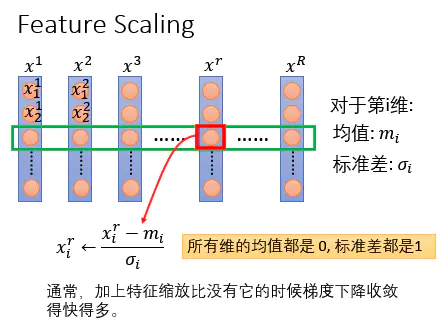## Internal Covariate Shift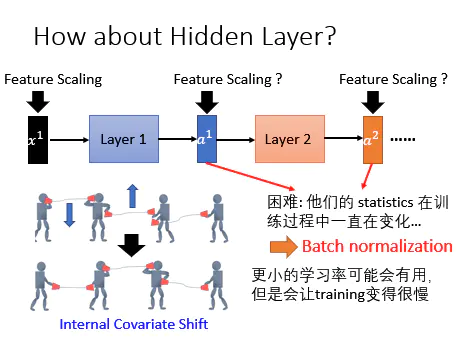## Batch Normalization原理

batch Normalization就是对每一个layer做Feature Scaling，就可以解决Internal Covariate Shift问题。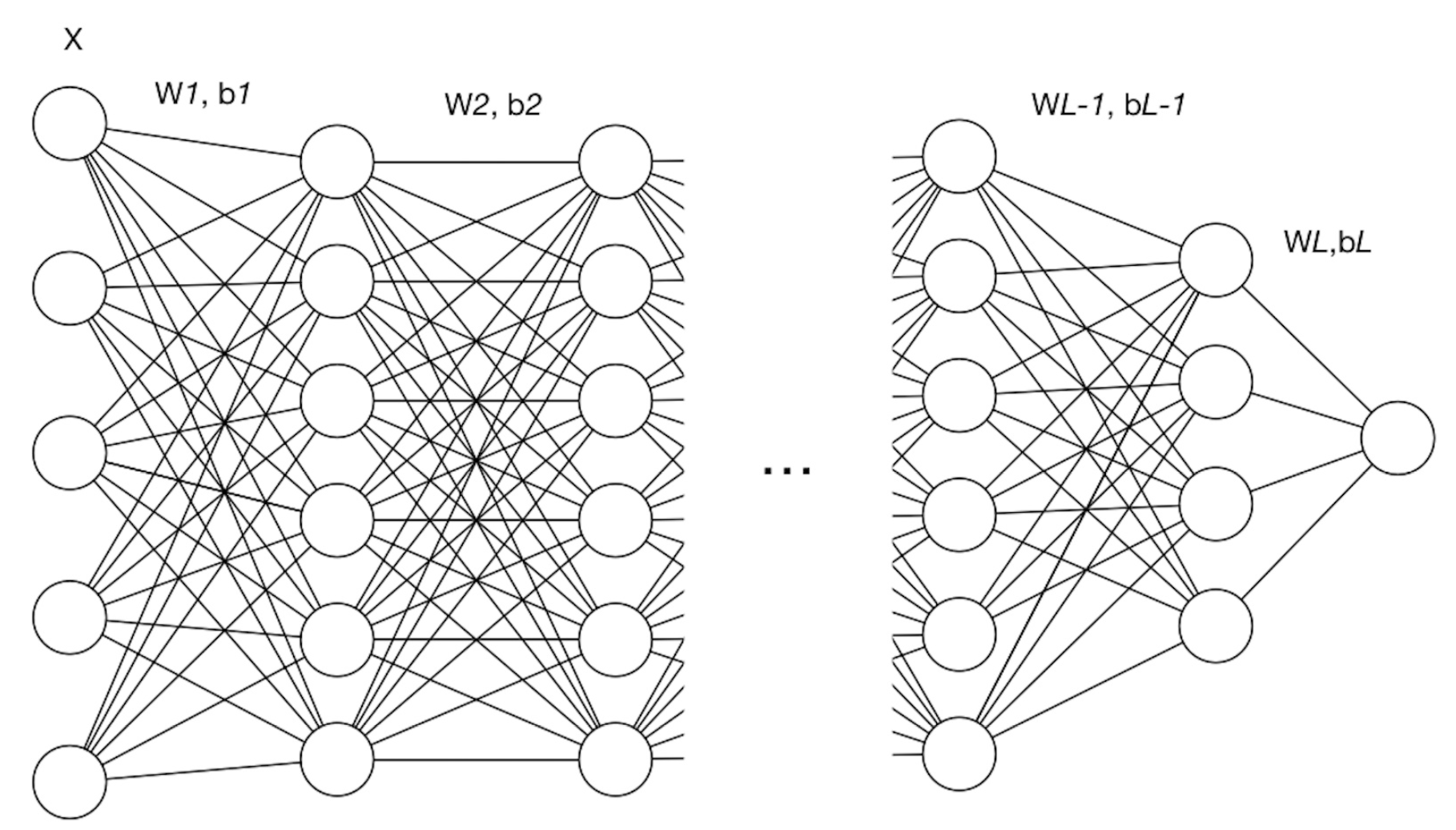• $l$ ：网络中的层标号

• $L$ ：网络中的最后一层或总层数

• $d_l$ ：第 $l$ 层的维度，即神经元结点数

• $W^{[l]}$ ：第 $l$ 层的权重矩阵， $W^{[l]} \in \R^{d_l\times d_{l-1}}$

• $b^{[l]}$ ：第 $l$ 层的偏置向量， $b^{l}\in \R^{d_l\times 1}$

• $Z^{[l]}$ ：第$l$ 层的线性计算结果，$Z^{[l]}=W^{[l]}\times input +b^{[l]}$

• $g^{[l]}(\cdot)$ ：第 $l$ 层的激活函数

• $A^{[l]}$ ：第 $l$ 层的非线性激活结果，$A^{[l]} = g^{[l]}(Z^{[l]})$

• $M$ ：训练样本的数量

• $N$ ：训练样本的特征数

• $X$ ：训练样本集，$\{X={x^{(1)},x^{(2)}, … ,x^{(M)}}\}$ （注意这里 $M$ 的一列是一个样本）

• $m$ ：batch size，即每个batch中样本的数量

• $X^{(i)}$：第 $i$ 个mini-batch的训练数据， $X=\{{x^{(1)},x^{(2)}, … ,x^{(k)}}\}$，其中 $X^{(i)}\in \R^{N\times m}$

$Z\in \R^{d_l\times m}$

$\mu_j = \frac1m\sum^m_{i=1}Z^{(i)}_j$

$\sigma^2_j = \frac 1m\sum^m_{i=1}(Z^{(i)}_j-\mu_j)^2$

$\hat Z_j=\frac{Z_j-\mu _j}{\sqrt{\sigma^2_j+\epsilon}}$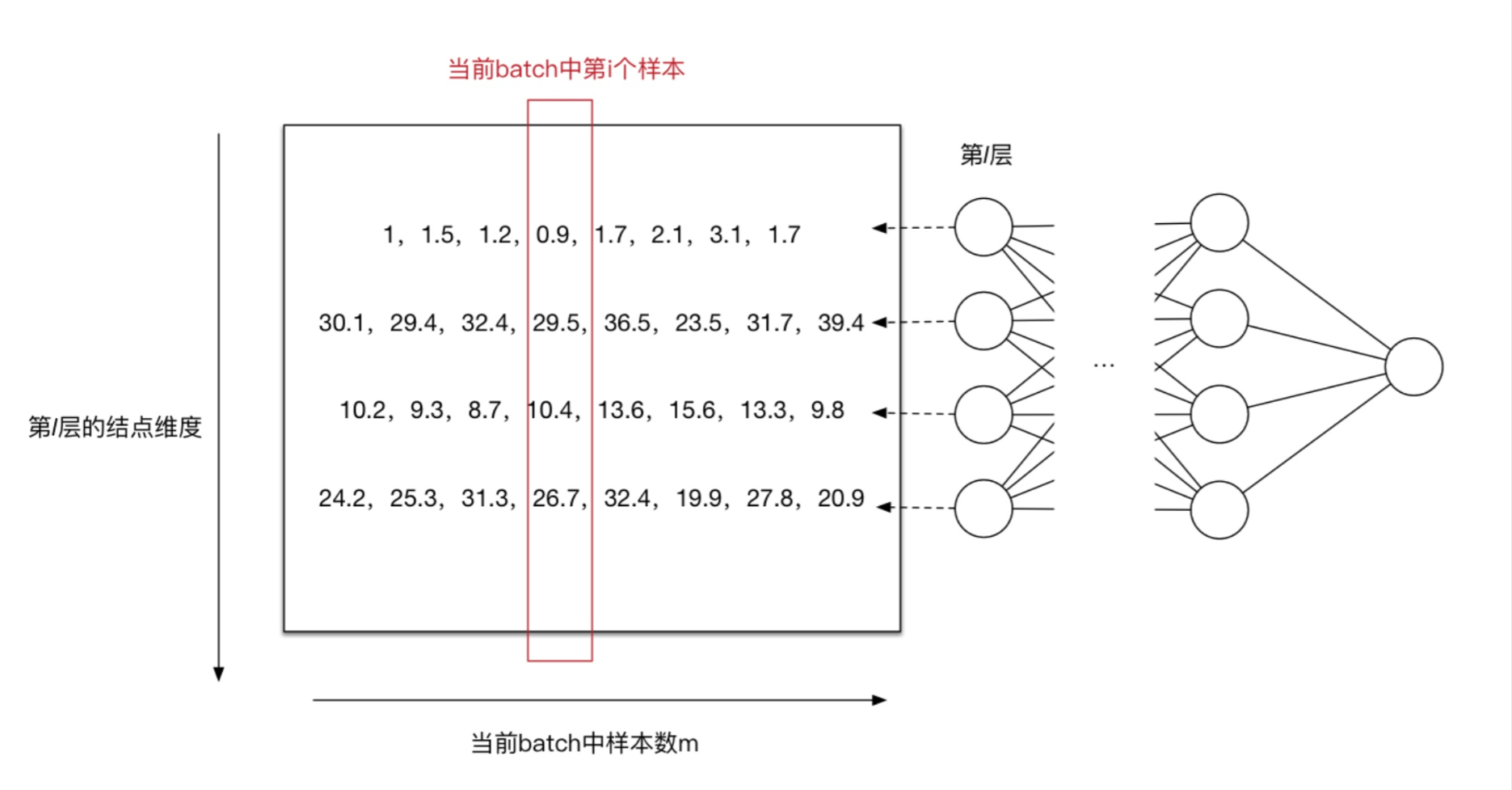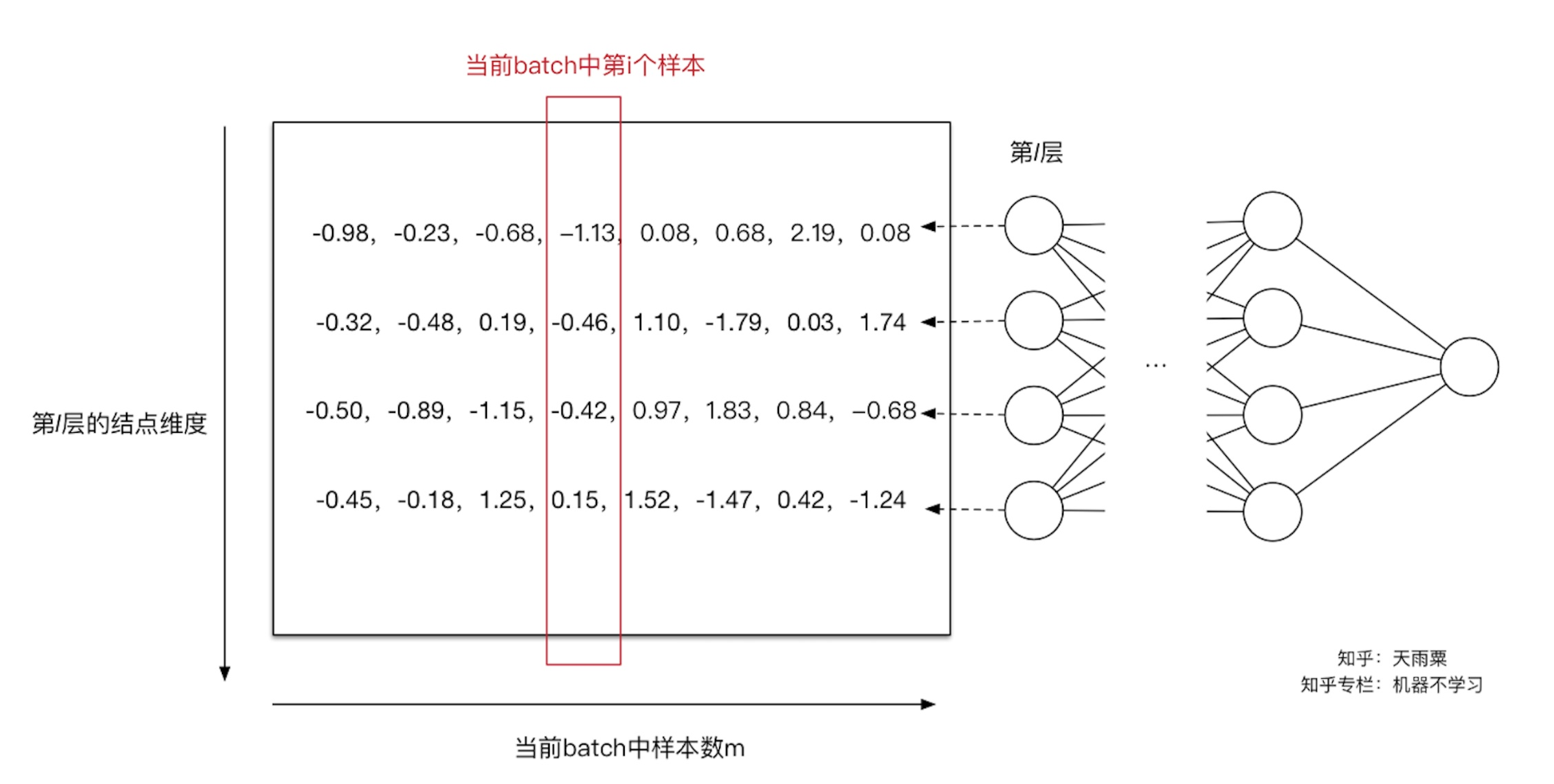Normalization操作虽然缓解了ICS（Internal Covariate Shift）问题，让每一层网络的输入数据分布都变得稳定，但却导致了数据表达能力的缺失。也就是通过变换操作改变了原有数据的信息表达（representation ability of the network），使得底层网络学习到的参数信息丢失。另一方面，通过让每一层的输入分布均值为0，方差为1，会使得输入在经过sigmoid或tanh激活函数时，容易陷入非线性激活函数的线性区域。## Batch Normalization的优势

• 解决了Internal Covariate Shift的问题：Internal Covariate Shift让学习率需要设很小，Batch Normalization以后学习率可以设大一点，所以training就快一点。

假设把 $W^1$ 都乘 $k$ 倍， $z$ 当然也就乘上 $k$ ，Normalization的时候， $\mu,\sigma$ 也是乘上 $k$.
分子乘 $k$ 倍，分母 $k$ 乘，做完Normalization以后没有变化。所以如果在initialize的时候， $W$ 的参数乘上 $k$ 倍，对它的output的结果是没有影响。